# 「MoreThanJava」Day 4：面向对象基础

LeetCode 89，因为题目晦涩而被点了1500+反对的搜索问题• 「MoreThanJava」 宣扬的是 「学习，不止 CODE」，本系列 Java 基础教程是自己在结合各方面的知识之后，对 Java 基础的一个总回顾，旨在 「帮助新朋友快速高质量的学习」
• 当然 不论新老朋友 我相信您都可以 从中获益。如果觉得 「不错」 的朋友，欢迎 「关注 + 留言 + 分享」，文末有完整的获取链接，您的支持是我前进的最大的动力！

# Part 1. 面向对象设计概述## 了解抽象## 什么是对象

### 对象像是一种模板### 对象的特征• 对象具有 标识 identity(每个对象都是独立的个体)
• 对象具有 状态 state(它具有各种可能会改变的属性)
• 对象具有 行为 behavior(它可以做事情，也可以让别人对它做事情)

## 编程语言中的抽象过程## 面向对象思想的突破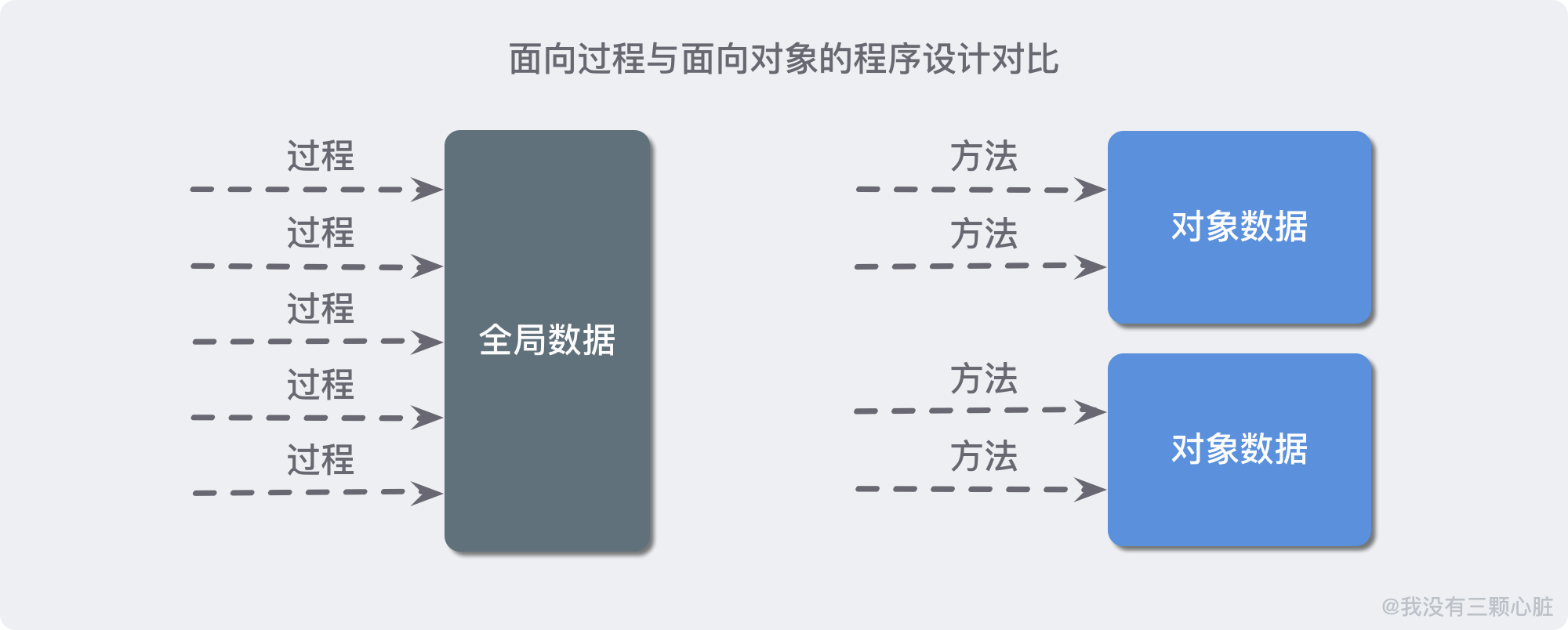## OOP 的起源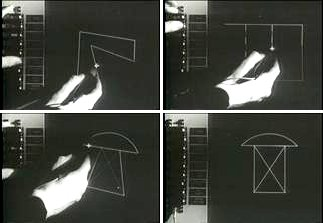## OOP 的规范化

Ivan 的项目和其他一些项目在 `1967` 年影响了 Simula 编程语言。该语言第一次直接将面向对象的思想引入到了 编程语言中 (重大更新之后被称为 `Simula-67`)

`1970` 年代，Xerox (负责鼠标和图形界面的发明) 在个人电脑上工作。他们希望通过操纵 GUI 和鼠标来创建任何人都可以轻松使用的计算机。## OOP 的普及化

• Objective-C 是 iOS 本机开发的原始语言。从那以后，Apple 对其进行了改进和增强，它仍然是 iOS 开发人员的常见选择。
• C ++ 是 C 编程语言的面向对象版本。C 和 C++ 仍被广泛使用，尤其是在非常专业的行业中。

• 部分影响来自于更清晰的表达复杂系统的方式；
• 也许最重要的原因 （也是从操作系统体系结构派生而来的） 是，当您给某人一个结构时，您很少希望他们拥有无限的特权。仅仅进行类型匹配甚至还不能满足需求。保护某些对象而不保护某些对象也不是非常合理有用。

# Part 2. 类与对象概述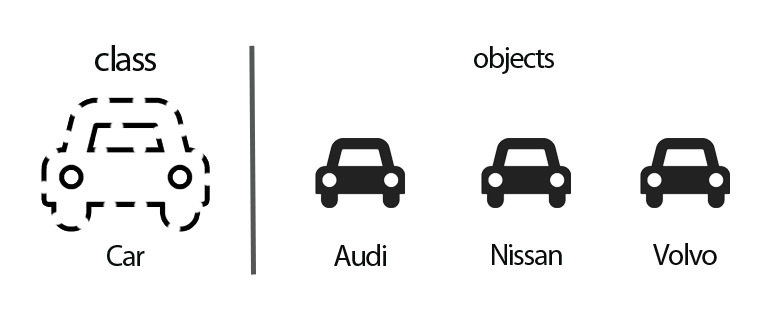## 定义类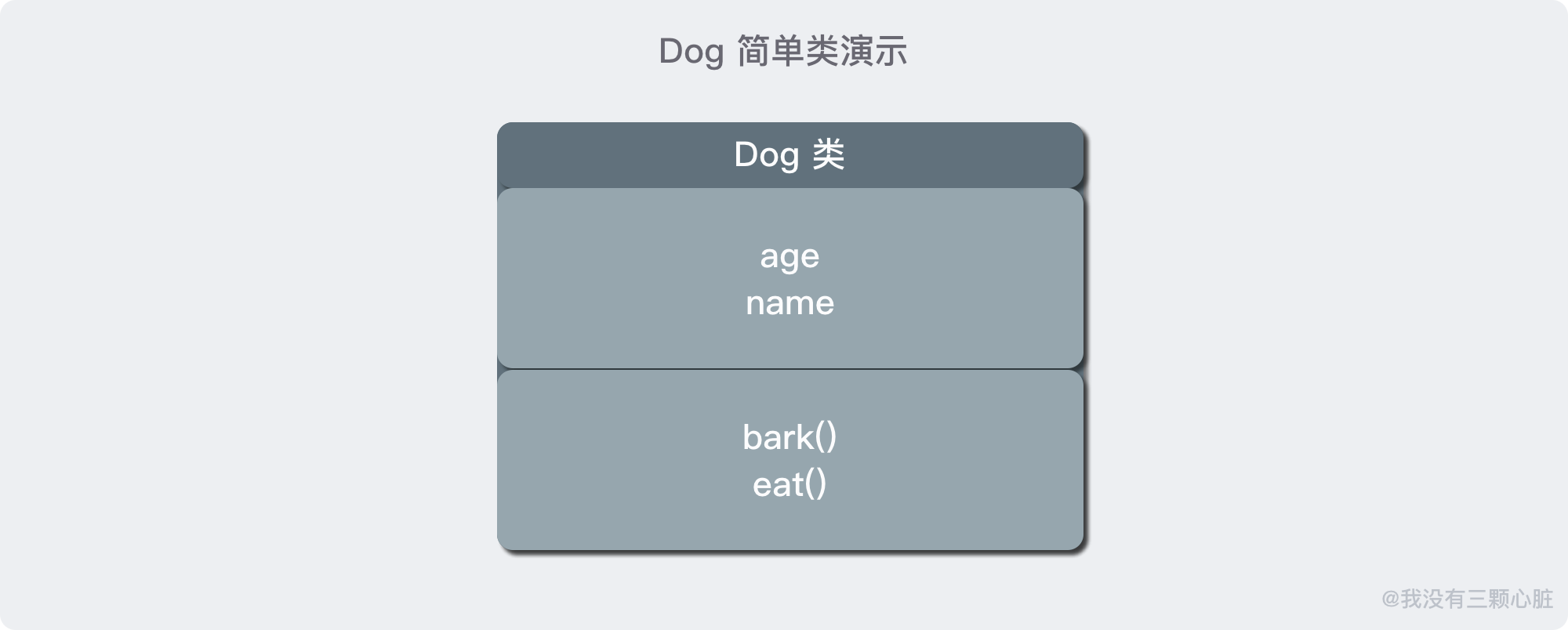``````public class Dog {

// 参数
private String name;
private Integer age;

// 构造器
public Dog(String name, Integer age) {
this.name = name;
this.age = age;
}

// 字段访问器
public String getName() {
return name;
}

// 字段访问器
public Integer getAge() {
return age;
}

// 方法 - 叫
void bark() {
System.out.println("汪汪汪！");
}

// 方法 - 吃东西
void eat() {
System.out.println("一只" + age + "岁大的名叫 " + name + " 的狗正在吃东西！");
}
}

``````

### 剖析 Dog 类

``````public Dog(String name, Integer age)
public String getName()
public Integer getAge()
public void bark()
public void eat()
``````

``````private String name;
private Integer age;
``````

### 从构造器开始

``````public Dog(String name, Integer age) {
this.name = name;
this.age = age;
}
``````

``````new Dog("大黄", 1)
``````

``````name = "大黄"
age = 1
``````

``````dogInstance.Dog("小黄", 2);  // ERROR
``````

• 构造器与类同名；
• 每个类可以有一个以上的构造器；
• 构造器可以有 `0` 个、`1` 个或多个参数；
• 构造器没有返回值；
• 构造器总是伴随着 `new` 操作符一起调用。

### 封装的优点

``````public String getName() {
return name;
}
public Integer getAge() {
return age;
}
``````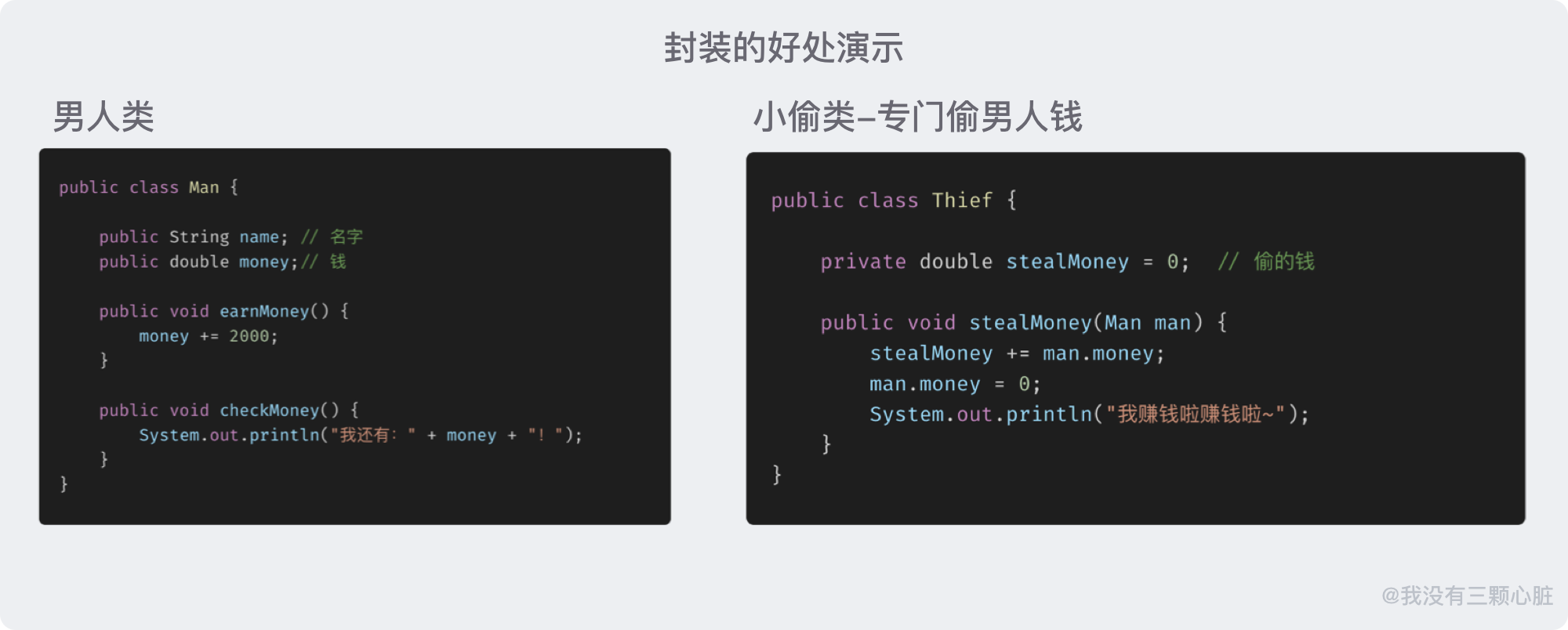``````public int age;
``````

``````instance.age = 10;
``````

## 创建和使用类

``````Dog dog = new Dog("大黄", 1);
``````

``````var dog = new Dog("大黄", 1);
``````

``````dog.eat();  // 调用该实例的 eat() 方法
``````

## Java 使用引用来操纵对象``````String s;
``````

``````String s = "abcd";
``````

### null 引用

``````String s = null;
System.out.println(s.length());  // NullPointException
``````

“宽容型” 方法 是把 `null` 参数转换为一个适当的非 `null` 值：

``````if (n == null) {
name = "unknow";
} else {
name = n;
}
``````

Java 9 中，`Objects`(JDK 自带的工具类) 对此提供了一个便利方法：

``````name = Objects.requireNonNullElse(n, "unknow");  // 效果与上面代码等同
``````

“严格型” 方法 则是干脆拒绝 `null` 参数：

``````name = Objects.requireNonNull(n, "The name cannot be null!");
``````

1. 异常报告会提供这个问题的描述；(也就是 `The name cannot be null!`)
2. 异常报告会准确地支出问题所在的位置，否则异常可能在其他地方出现，而很难追踪到真正导致问题的这个构造器参数；

# Part 3. 面向对象的四大特性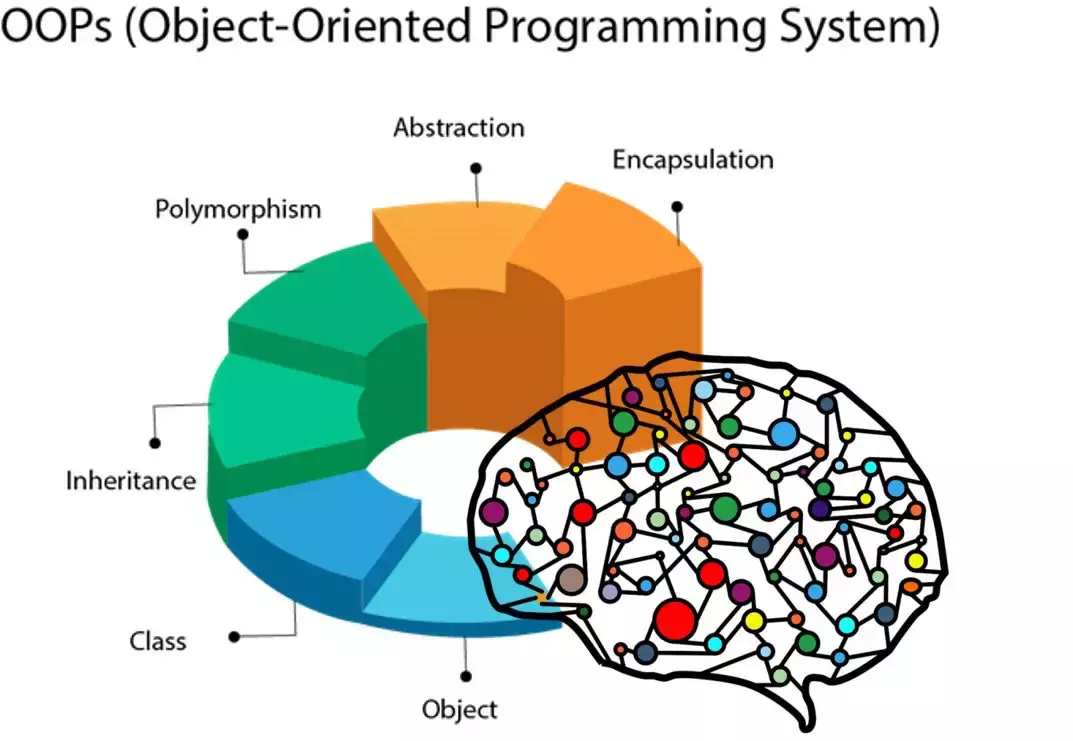(关于继承和多态会在后续章节里面详细说明, 这里只作简单描述用于简单理解..)

## 封装## 继承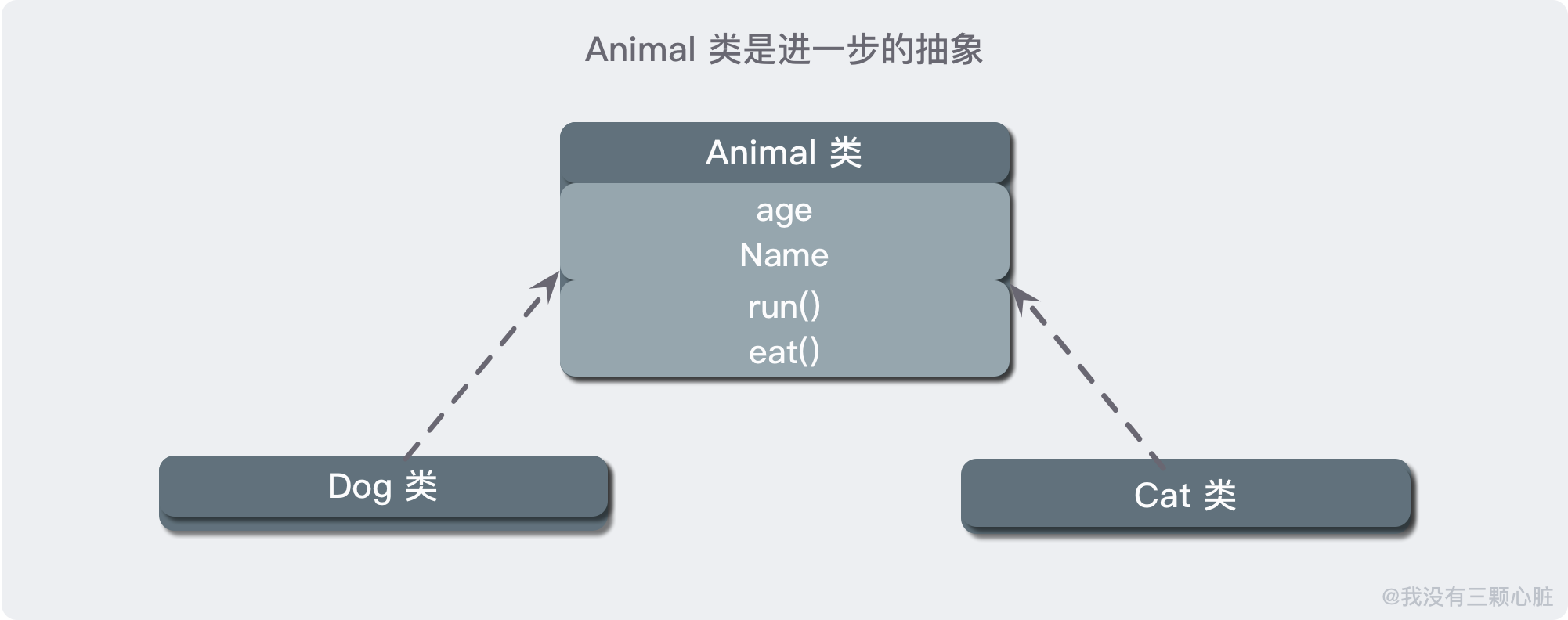1. 继承是一种 强耦合 的关系，父类如果做出一定改变，那么子类也必然会改变；
2. 继承 破坏了封装，对于子类而言，它的实现对子类来说都是透明的；

## 多态(完)

# 要点回顾

1. 类和对象 – 什么是类 / 什么是对象 / OOP 起源和发展 / 面向对象其他相关概念
2. 定义类 – 基本结构 / 属性和方法 / 构造器
3. 使用对象 – 创建对象 / 给对象发消息
4. 面向对象的四大支柱 – 抽象 / 封装 / 继承 / 多态的简单介绍
5. 基础练习 – 定义 Dog 类 / 定义时钟类 / 定义图形类 (下方)

# 练习

## 练习 1：定义一个类描述数字时钟

``````public class Clock {

private Integer hour;
private Integer minute;
private Integer second;

public Clock(Integer hour, Integer minute, Integer second) {
this.hour = hour;
this.minute = minute;
this.second = second;
}

/**
* 时钟走字(走1s)
*/
public void run() {
second += 1;
if (second.equals(60)) {
second = 0;
minute += 1;
if (minute.equals(60)) {
minute = 0;
hour += 1;
if (hour.equals(24)) {
hour = 0;
}
}
}
}

/**
* 显示当前时间
* @return
*/
public String showCurrentTime() {
return String.format("当前时间是：%d时:%d分:%d秒", hour, minute, second);
}

/**
* 内部测试
* @throws InterruptedException - 使用 Thread.sleep() 需要手动检测该异常, 这里节约篇幅直接抛出
*/
public static void main(String[] args) throws InterruptedException {
Clock clock = new Clock(23, 59, 58);
while (true) {
clock.run();
System.out.println(clock.showCurrentTime());
// 让当前线程睡 1s
}
}
}
``````

## 练习 2：定义一个类描述平面上的点并提供移动点和计算到另一个点距离的方法

``````public class Point {

private Integer x;
private Integer y;

public Point() {
this.x = 0;
this.y = 0;
}

public Point(Integer x, Integer y) {
this.x = x;
this.y = y;
}

/**
* 移动到指定位置
*/
public void moveTo(Integer x, Integer y) {
this.x = x;
this.y = y;
}

/**
* 移动指定的距离
*/
public void moveBy(Integer dx, Integer dy) {
this.x += dx;
this.y += dy;
}

/**
* 计算并返回与另一个点的距离
*/
public Double distanceTo(Point other) {
int dx = this.x - other.x;
int dy = this.y - other.y;
return Math.sqrt(dx ^ 2 + dy ^ 2);
}

/**
* 当前的坐标信息
*/
public String currentLocation() {
return String.format("当前点横坐标：%d，纵坐标：%d", x, y);
}

/**
* 内部测试
*/
public static void main(String[] args) {
Point point1 = new Point(3, 5);
Point point2 = new Point();

System.out.println(point1.currentLocation());
System.out.println(point2.currentLocation());

point2.moveTo(-1, 2);
System.out.println(point2.currentLocation());

System.out.println(point1.distanceTo(point2));
}
}
``````

# 参考资料

1. 《Java 核心技术 卷 I》
2. 《Java 编程思想》
3. 《码出高效 Java 开发手册》
4. Deepen your knowledge by learning Object Oriented Programming (OOP) with Swift – https://openclassrooms.com/en/courses/4542221-deepen-your-knowledge-by-learning-object-oriented-programming-oop-with-swift
5. Think like a computer: the logic of programming – https://openclassrooms.com/en/courses/5261196-think-like-a-computer-the-logic-of-programming
6. Introduction to Computer Science using Java – http://programmedlessons.org/Java9/index.html#part02
7. Python 100 天从新手到大师 – https://github.com/jackfrued/Python-100-Days
• 本文已收录至我的 Github 程序员成长系列 【More Than Java】，学习，不止 Code，欢迎 star：https://github.com/wmyskxz/MoreThanJava
• 个人公众号 ：wmyskxz，个人独立域名博客：wmyskxz.com，坚持原创输出，下方扫码关注，2020，与您共同成长！「MoreThanJava」Day 4：面向对象基础